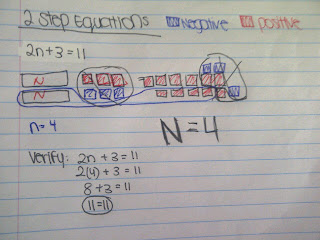## Sunday, May 8, 2011

### Lara's Algebra Post

Algebra: Writing a mathematical equation using a Letter.

Example : x + 4 = 5

Variable - a letter

that represents

a number in an algebraic equation or expression
Constant - the integer in an algebraic expression or equation

Expression - pattern, many

Equation - only has one answer, has an equal sign ex. 5x=25, 5x5=25

Solving an Algebraic equation.

Steps:

1. Isolate the variable.

2. Cancel using the opposite of

the number.

3. Constant is cancelled with the zero pair.

4. Balance, do the same

operation on the other side of the = sign.

5. Verify to check